Last updated: 2019-01-09

This reproducible R Markdown analysis was created with workflowr (version 1.1.1.9001). The Report tab describes the reproducibility checks that were applied when the results were created. The Past versions tab lists the development history.

R Markdown file: up-to-date

Great! Since the R Markdown file has been committed to the Git repository, you know the exact version of the code that produced these results.

Environment: empty

Great job! The global environment was empty. Objects defined in the global environment can affect the analysis in your R Markdown file in unknown ways. For reproduciblity it’s best to always run the code in an empty environment.

Seed: set.seed(12345)

The command set.seed(12345) was run prior to running the code in the R Markdown file. Setting a seed ensures that any results that rely on randomness, e.g. subsampling or permutations, are reproducible.

Session information: recorded

Great job! Recording the operating system, R version, and package versions is critical for reproducibility.

Repository version: f440a87

Great! You are using Git for version control. Tracking code development and connecting the code version to the results is critical for reproducibility. The version displayed above was the version of the Git repository at the time these results were generated.

Note that you need to be careful to ensure that all relevant files for the analysis have been committed to Git prior to generating the results (you can use wflow_publish or wflow_git_commit). workflowr only checks the R Markdown file, but you know if there are other scripts or data files that it depends on. Below is the status of the Git repository when the results were generated:

Ignored files:
Ignored:    .DS_Store
Ignored:    .Rhistory
Ignored:    .Rproj.user/
Ignored:    figure/.DS_Store
Ignored:    figure/ch02/.DS_Store

Untracked files:
Untracked:  audition-extra.md
Untracked:  audition-figure/
Untracked:  audition.md
Untracked:  code/counts_per_sample.txt
Untracked:  code/table-s1.txt
Untracked:  data/counts_per_sample.txt
Untracked:  data/test.rds
Untracked:  docs/figure/alternative.Rmd/
Untracked:  docs/figure/ch04.Rmd/
Untracked:  factorial-dox.rds
Untracked:  notes.md



Note that any generated files, e.g. HTML, png, CSS, etc., are not included in this status report because it is ok for generated content to have uncommitted changes.

These are the previous versions of the R Markdown and HTML files. If you’ve configured a remote Git repository (see ?wflow_git_remote), click on the hyperlinks in the table below to view them.

File Version Author Date Message
html f440a87 John Blischak 2018-08-20 Build site.
html 4976490 John Blischak 2018-08-20 Build site.
Rmd 1920833 John Blischak 2018-08-20 Refactor first edition of chapter 1 into distinct lessons.

Introduction

The simulation and visualizations below demonsrate the differences in the results due to limma sharing information across genes to shrink the estimates of the variance.

Setup

library("cowplot")
library("dplyr")
library("ggplot2")
theme_set(theme_classic(base_size = 16))
library("knitr")
opts_chunk$set(fig.width = 10, fig.height = 5, message = FALSE) library("stringr") library("tidyr") Simulation Create some synthetic data for illustrating concepts. The simulated gene expression matrix has 100 genes and 6 samples (3 treatment and 3 control). set.seed(12345) create_exp_mat <- function(n1, n2, ng, alpha_mean, beta_mean, epsilon_sd) { status <- c(rep(0, n1), rep(1, n2)) ns <- length(status) status <- matrix(status, nrow = 1) alpha <- rnorm(ng, mean = alpha_mean, sd = 1) beta <- matrix(rnorm(ng, mean = beta_mean, sd = 1), ncol = 1) epsilon <- matrix(rnorm(ng * ns, mean = 0, sd = epsilon_sd), nrow = ng, ncol = ns) Yg <- alpha + beta %*% status + epsilon return(Yg) } gexp <- rbind( # 30 non-DE genes with high variance create_exp_mat(n1 = 3, n2 = 3, ng = 30, alpha_mean = 10, beta_mean = -1:1, epsilon_sd = 3), # 30 non-DE genes with low variance create_exp_mat(n1 = 3, n2 = 3, ng = 30, alpha_mean = 10, beta_mean = -1:1, epsilon_sd = 1), # 10 upregulated DE genes with low variance create_exp_mat(n1 = 3, n2 = 3, ng = 10, alpha_mean = 10, beta_mean = 5, epsilon_sd = 1), # 10 upregulated DE genes with high variance create_exp_mat(n1 = 3, n2 = 3, ng = 10, alpha_mean = 10, beta_mean = 5, epsilon_sd = 3), # 10 downregulated DE genes with low variance create_exp_mat(n1 = 3, n2 = 3, ng = 10, alpha_mean = 10, beta_mean = -5, epsilon_sd = 1), # 10 downregulated DE genes with high variance create_exp_mat(n1 = 3, n2 = 3, ng = 10, alpha_mean = 10, beta_mean = -5, epsilon_sd = 3) ) # Add names for samples group <- rep(c("con", "treat"), each = ncol(gexp) / 2) samples <- paste0(group, 1:3) colnames(gexp) <- samples # Add names for genes genes <- sprintf("gene%02d", 1:nrow(gexp)) rownames(gexp) <- genes heatmap(gexp)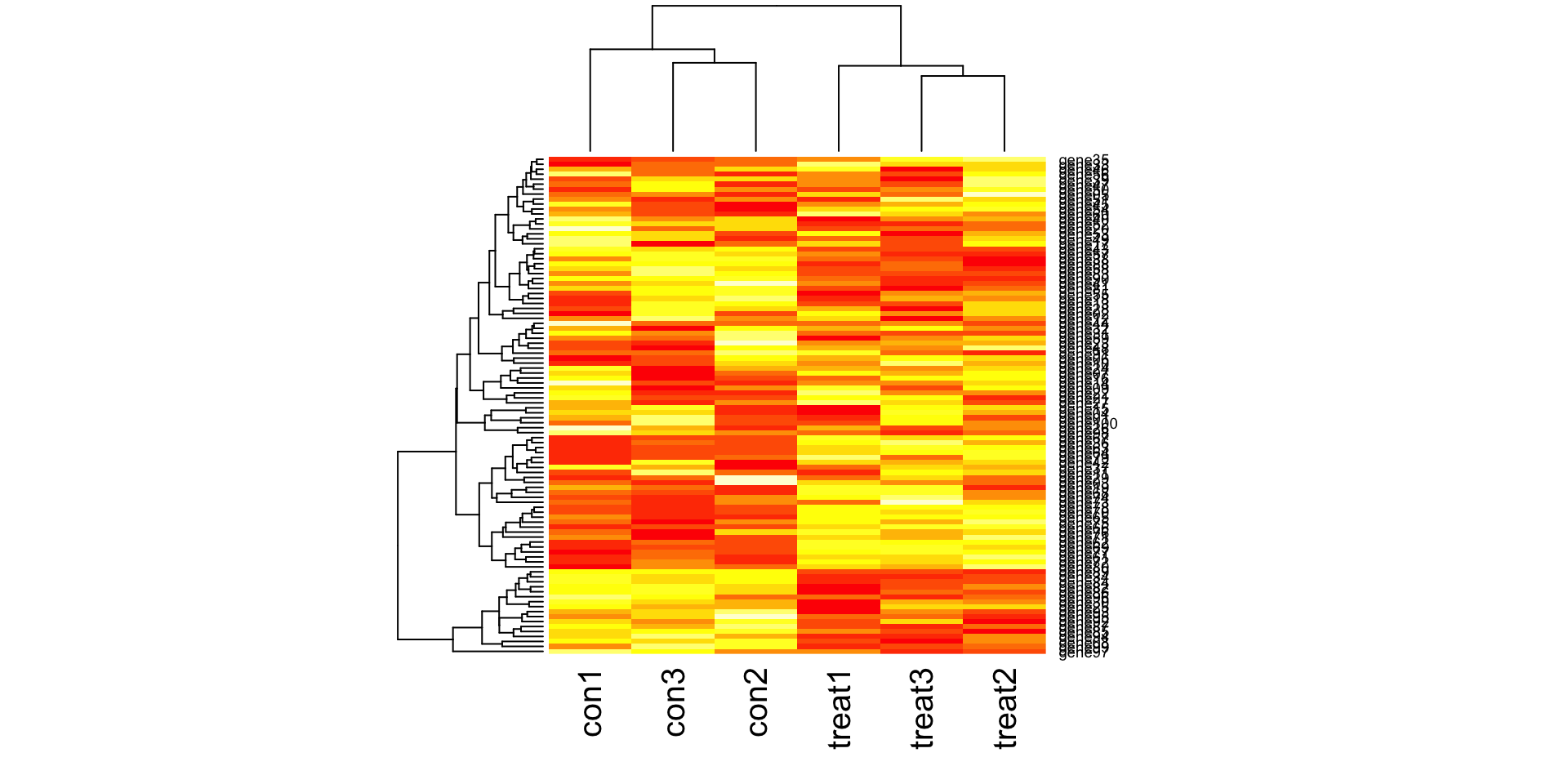Version Author Date 4976490 John Blischak 2018-08-20 Standard linear model Find differentially expressed genes using a standard linear model. lm_beta <- numeric(length = nrow(gexp)) lm_se <- numeric(length = nrow(gexp)) lm_p <- numeric(length = nrow(gexp)) for (i in 1:length(lm_p)) { mod <- lm(gexp[i, ] ~ group) result <- summary(mod) lm_beta[i] <- result$coefficients[2, 1]
lm_se[i] <- result$coefficients[2, 2] lm_p[i] <- result$coefficients[2, 4]
}
hist(lm_p, xlab = "p-values", main = "Standard linear model")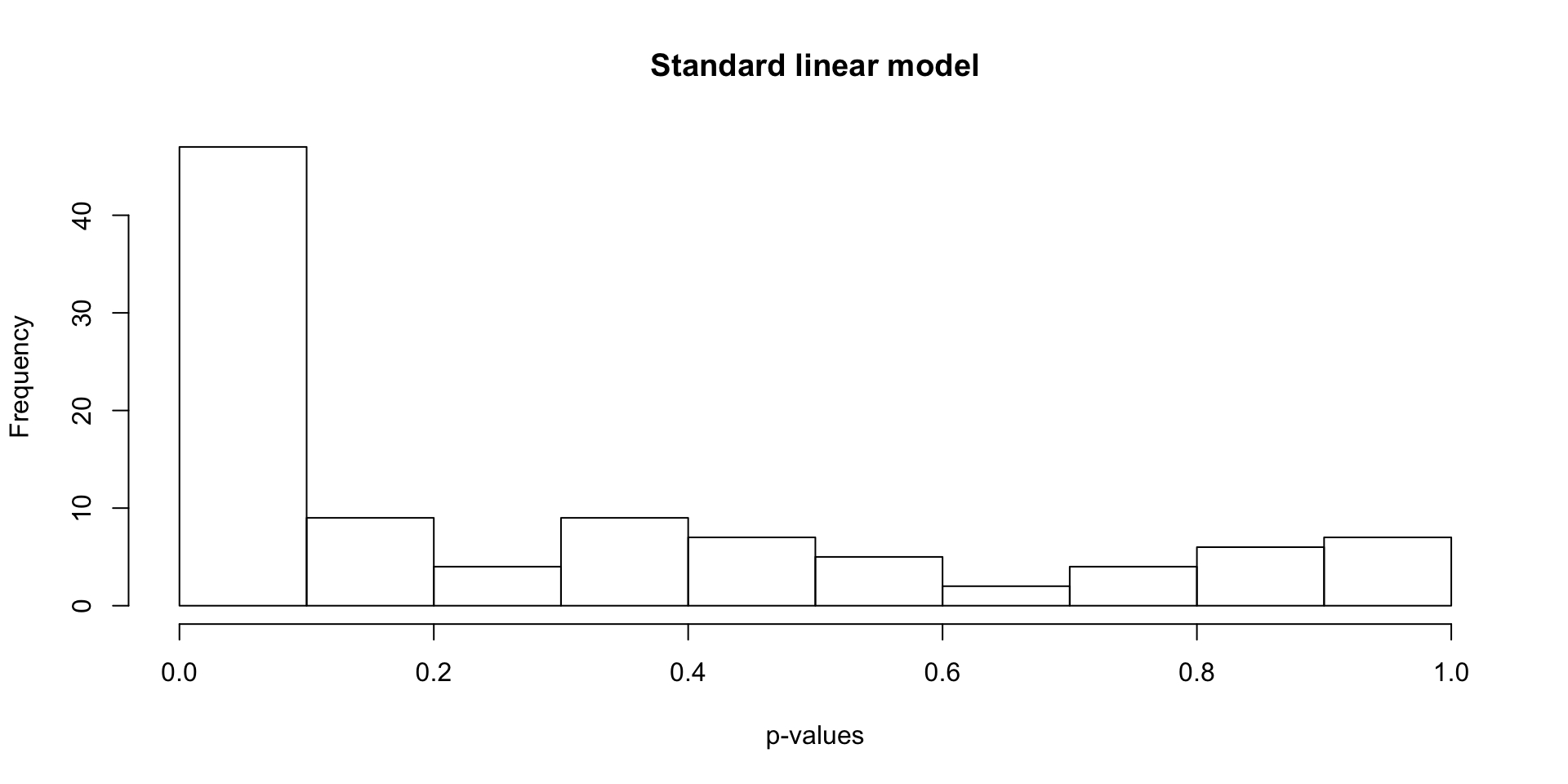Version Author Date
4976490 John Blischak 2018-08-20

limma linear model

Find differentially expressed genes using limma.

library("limma")
design <- model.matrix(~group)
colnames(design) <- c("Intercept", "treat")
fit <- lmFit(gexp, design)
head(fit$coefficients)  Intercept treat gene01 11.316083 -2.4577980 gene02 9.833304 2.7130980 gene03 12.653098 -0.2048963 gene04 12.275601 0.2934781 gene05 8.617135 2.3383110 gene06 5.878178 3.9361382 fit <- eBayes(fit) results <- decideTests(fit[, 2]) summary(results)  treat -1 15 0 71 1 14 stats <- topTable(fit, coef = "treat", number = nrow(fit), sort.by = "none") hist(stats[, "P.Value"], xlab = "p-values", main = "limma linear model")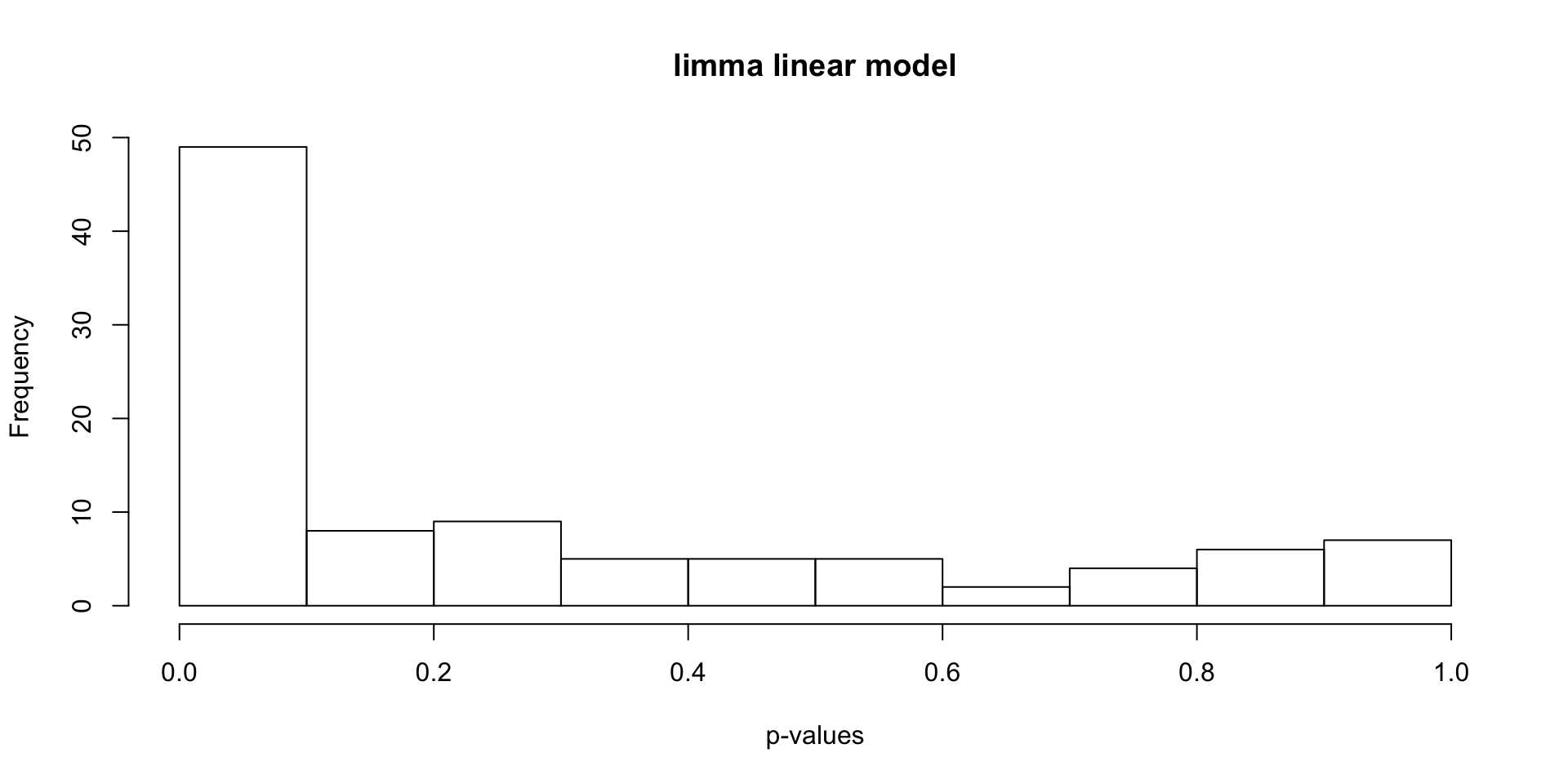Version Author Date 4976490 John Blischak 2018-08-20 Comparison Compare the p-values from lm and limma (both adjusted for multiple testing with the BH FDR). stats <- cbind(stats, sd = apply(gexp, 1, sd), var = apply(gexp, 1, var), lm_beta, lm_se, lm_p = p.adjust(lm_p, method = "BH")) stats$labels_pre <- c(rep("non-DE; high-var", 30),
rep("non-DE; low-var", 30),
rep("DE-up; low-var", 10),
rep("DE-up; high-var", 10),
rep("DE-down; low-var", 10),
rep("DE-down; high-var", 10))

stats$labels <- rep("non-DE", nrow(stats)) stats$labels[stats$adj.P.Val < 0.05 & stats$lm_p < 0.05] <- "DE"
stats$labels[stats$adj.P.Val < 0.05 & stats$lm_p >= 0.05] <- "limma-only" stats$labels[stats$adj.P.Val >= 0.05 & stats$lm_p < 0.05] <- "lm-only"
table(stats$labels)  DE limma-only lm-only non-DE 22 7 3 68  table(stats$labels, stats$labels_pre)  DE-down; high-var DE-down; low-var DE-up; high-var DE 0 7 3 limma-only 1 3 1 lm-only 0 0 0 non-DE 9 0 6 DE-up; low-var non-DE; high-var non-DE; low-var DE 9 0 3 limma-only 1 1 0 lm-only 0 0 3 non-DE 0 29 24 stopifnot(stats$logFC == stats$lm_beta) de <- data.frame(effect_size = stats$lm_beta,
std_dev = stats$sd, lm = stats$lm_p < 0.05,
limma = stats$adj.P.Val < 0.05) head(de)  effect_size std_dev lm limma 1 -2.4577980 3.750555 FALSE FALSE 2 2.7130980 3.353835 FALSE FALSE 3 -0.2048963 1.715542 FALSE FALSE 4 0.2934781 3.511502 FALSE FALSE 5 2.3383110 1.955378 FALSE FALSE 6 3.9361382 3.326517 FALSE FALSE # View the number of discrepancies table(de$lm, de$limma)  FALSE TRUE FALSE 68 7 TRUE 3 22 # Plot effect size (y-axis) vs. standard deviation (x-axis) ggplot(de, aes(x = std_dev, y = effect_size, color = limma)) + geom_point()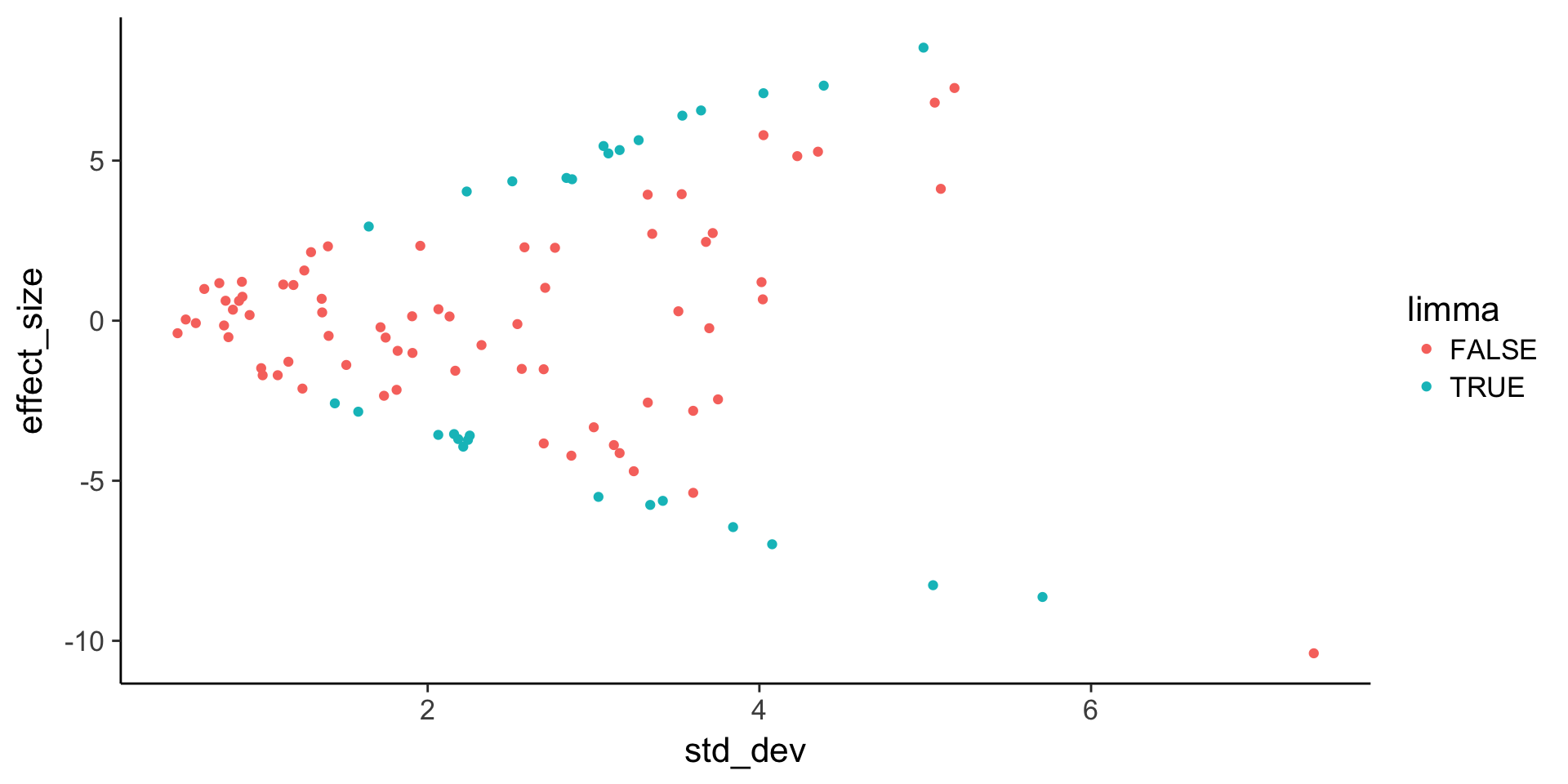Version Author Date 4976490 John Blischak 2018-08-20 ggplot(stats, aes(x = sd, y = logFC, color = labels)) + geom_point()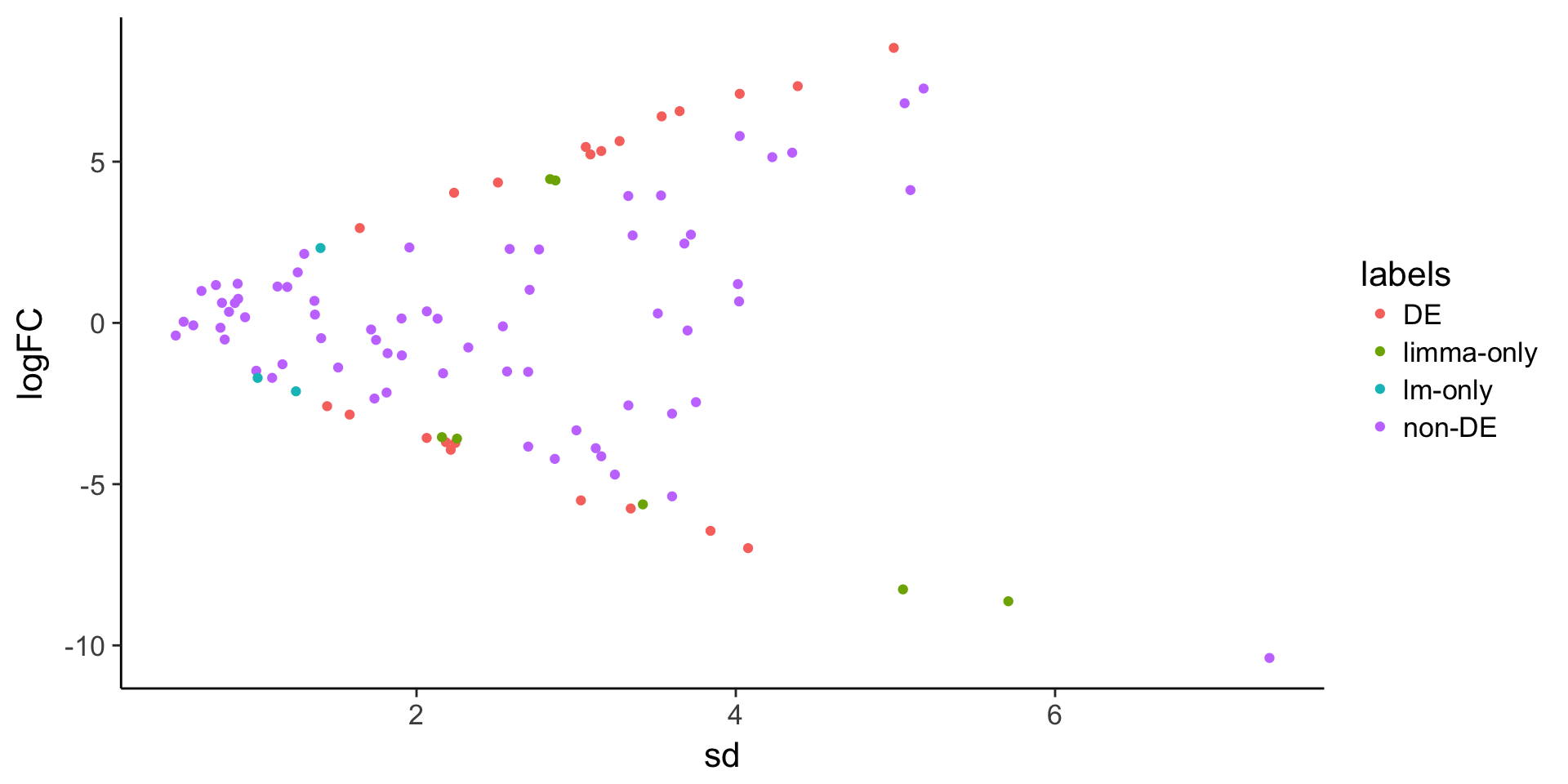Version Author Date 4976490 John Blischak 2018-08-20 ggplot(stats, aes(x = logFC, y = -log10(P.Value), color = labels)) + geom_point()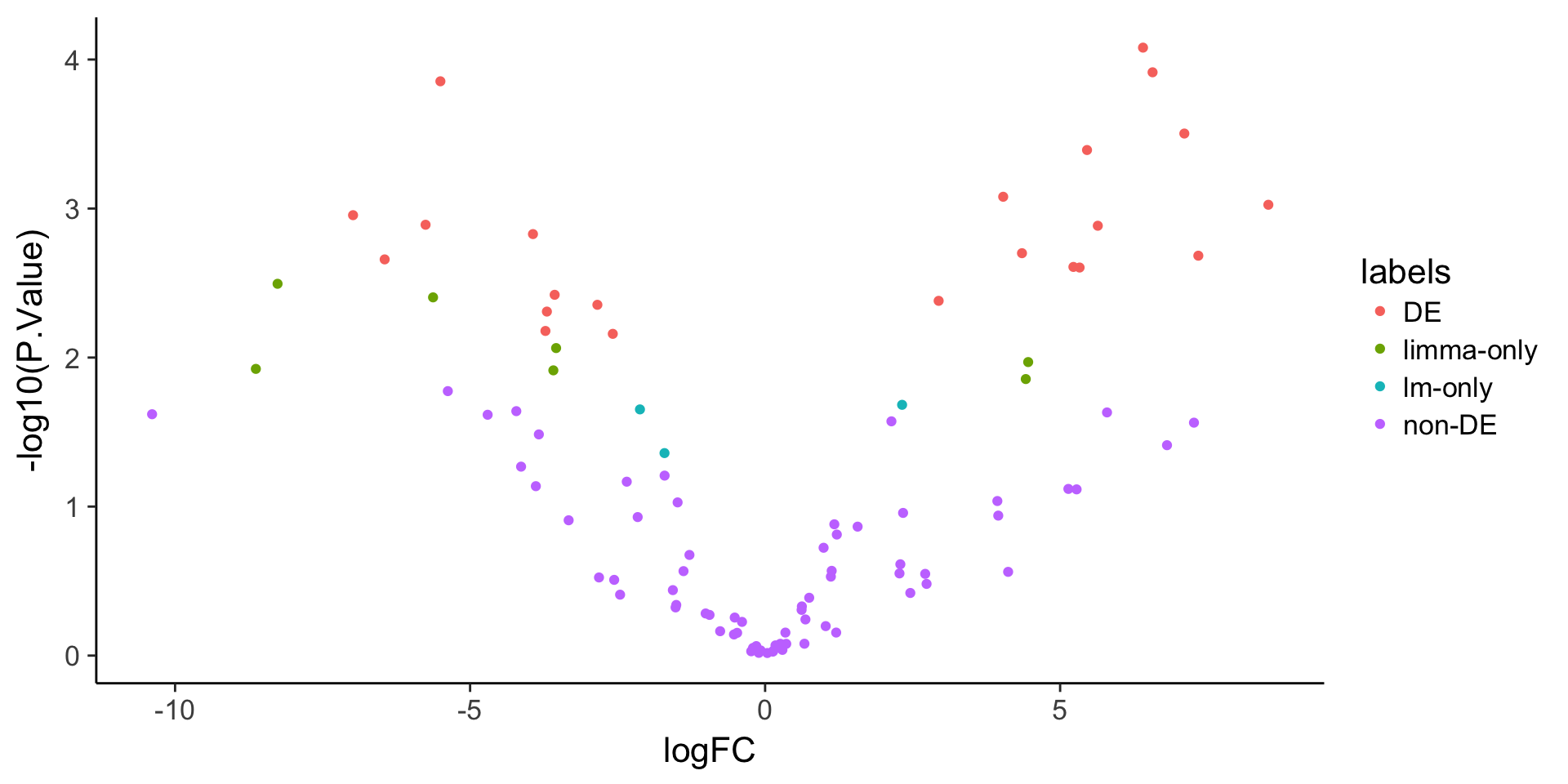Version Author Date 4976490 John Blischak 2018-08-20 Example genes Visualize example genes with boxplots. Note that the limma-only gene has higher variance compared to the lm-only gene. # Find a good example of a DE gene index <- which(stats$labels_pre == "DE-up; low-var" & stats$labels == "DE") single_gene <- gexp %>% as.data.frame %>% slice(index) %>% gather(key = "group", value = "gene") %>% mutate(group = str_extract(group, "[a-z]*")) %>% as.data.frame() # Find a gene that is DE for both, DE for lm-only, and DE for limma-only de_not <- de_lm <- which(stats$labels == "non-DE" &
stats$labels_pre == "non-DE; high-var" & stats$logFC > 0)
de_both <- which(stats$labels == "DE" & stats$labels_pre == "DE-up; low-var")
de_lm <- which(stats$labels == "lm-only" & stats$labels_pre == "non-DE; low-var" &
stats$logFC > 0) de_limma <- which(stats$labels == "limma-only" &
stats\$labels_pre == "DE-up; high-var")

compare <- gexp %>%
as.data.frame() %>%
slice(c(de_not, de_both, de_lm, de_limma)) %>%
mutate(type = c("neither", "both", "lm-only", "limma-only")) %>%
gather(key = "group", value = "gene", con1:treat3) %>%
mutate(group = str_extract(group, "[a-z]*")) %>%
as.data.frame()

head(compare)
        type group      gene
1    neither   con  6.681872
2       both   con  8.555641
3    lm-only   con  9.959914
4 limma-only   con 11.391149
5    neither   con  8.144218
6       both   con  7.977472
# Plot gene expression (gene; y-axis) vs. group (x-axis)
ggplot(compare, aes(x = group, y = gene)) +
geom_boxplot() +
facet_wrap(~type, nrow = 1)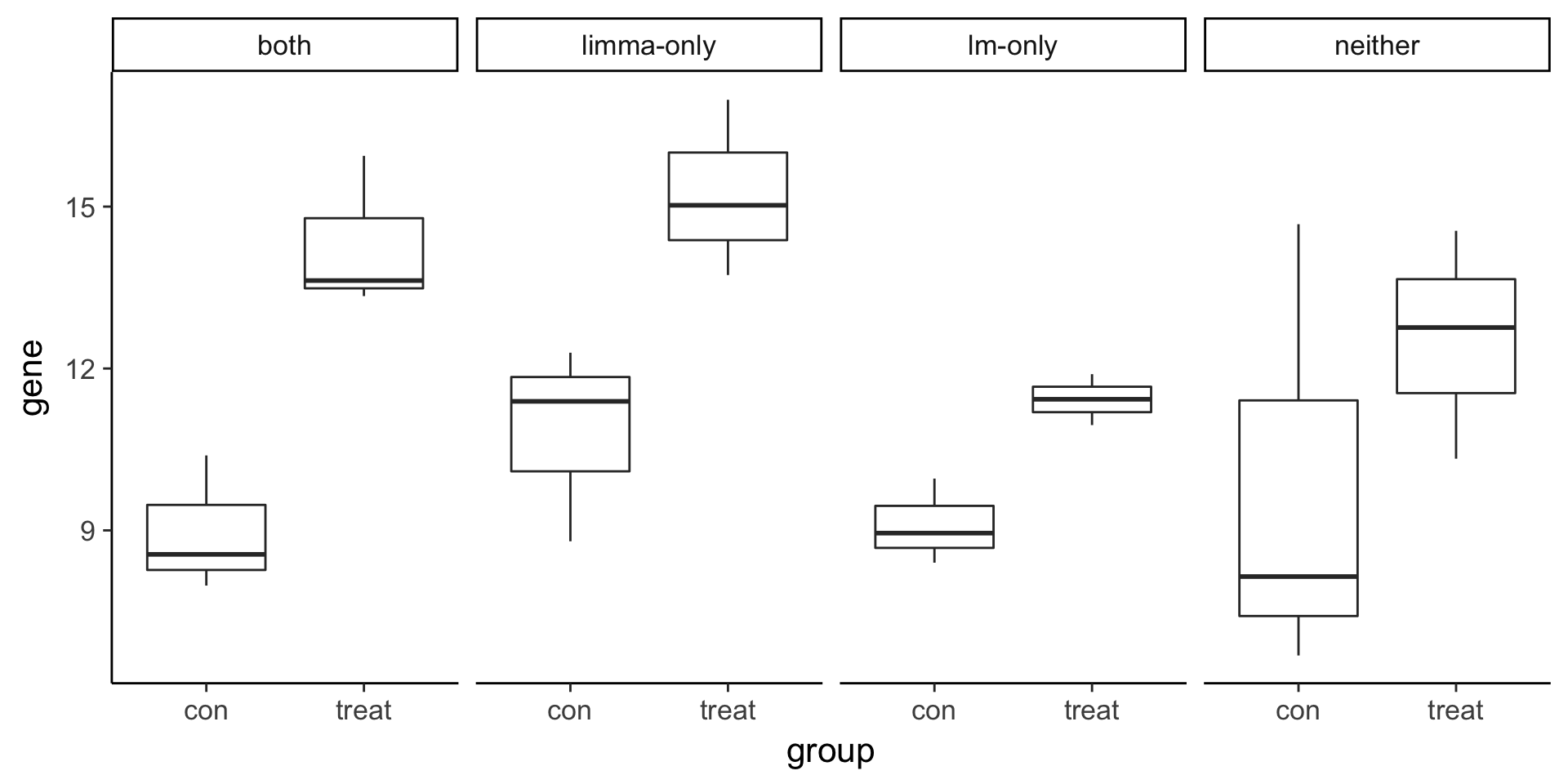Version Author Date
4976490 John Blischak 2018-08-20

sessionInfo()
R version 3.3.3 (2017-03-06)
Platform: x86_64-apple-darwin13.4.0 (64-bit)
Running under: OS X Yosemite 10.10.5

locale:
 en_US.UTF-8/en_US.UTF-8/en_US.UTF-8/C/en_US.UTF-8/en_US.UTF-8

attached base packages:
 stats     graphics  grDevices utils     datasets  methods   base

other attached packages:
 bindrcpp_0.2.2 limma_3.30.13  tidyr_0.8.1    stringr_1.3.1
 knitr_1.20     dplyr_0.7.7    cowplot_0.9.2  ggplot2_2.2.1

loaded via a namespace (and not attached):
 Rcpp_1.0.0           bindr_0.1.1          whisker_0.3-2
 magrittr_1.5         workflowr_1.1.1.9001 htmldeps_0.1.1
 tidyselect_0.2.3     munsell_0.5.0        colorspace_1.3-2
 R6_2.2.2             rlang_0.3.0.1        plyr_1.8.4
 tools_3.3.3          grid_3.3.3           gtable_0.2.0
 git2r_0.23.0         htmltools_0.3.6      assertthat_0.2.0
 yaml_2.2.0           lazyeval_0.2.1       rprojroot_1.3-2
 digest_0.6.13        tibble_1.4.2         purrr_0.2.5
 fs_1.2.6             glue_1.2.0.9000      evaluate_0.10.1
 rmarkdown_1.10.14    labeling_0.3         stringi_1.2.3
 pillar_1.2.2         scales_0.5.0         backports_1.1.2
 pkgconfig_2.0.1